# Math SprintsMath Sprints Workbooks 1 through 6 can be used to develop student’s mental math skills in conjunction with Singapore Math’s Primary Mathematics or most other math programs. Exercises require students to think quickly, but they will also write answers on the worksheets within a limited time. The Introduction to each book explains the research that shows the need for automaticity in using basic math facts as well as the value of timed exercises.

Each workbook might be used over a range of grade levels. For example, the publisher suggests Math Sprints Workbook 4 for grades four and five, but it might also be used with a sixth grader if that seems more appropriate.

Math Sprints will generally be used once a week since there are 29 Sprints per workbook. However, there are two options—A and B—for each Sprint, and if you use both that doubles the number. The A Sprint is a little easier than the B Sprint for each lesson, but both are working on essentially the same concept. For example, one A Sprint has the problem 90 – 9 while the B Sprint has 390 – 309 on the corresponding worksheet. Interestingly, Sprints were designed so that the answers are the same for Sprints A and B. This allows a classroom teacher to adapt the level of difficulty within the classroom, while making it easier to check the answers. An answer key is at the back of each book.

Each sprint is also divided into two parts. Students are given one minute to solve as many of the problems as they can on the first half. The first half is scored. Children take a stretch break and then tackle the second half, trying to improve their score over the first half. The idea is to get students to continually try to better their own scores without concern for how other students are doing. There are more problems than can be solved in the allotted time, but you can adjust the time if needed. The author says that it is important to maintain a sense of urgency, so you generally do not want to allow time for all problems to be solved.

Problems on each Sprint—both parts as well as versions A and B—all focus on a single math concept such as subtracting two digit numbers, identifying greater than, less than, and equal relationships; multiplying two numbers; simplifying fractions; or rounding numbers. There are very few word problems—none in some of the workbooks. The number of problems per Sprint varies by the type of Sprint since some types of problems will take more time to solve.

For homeschoolers, students should work directly in the book. Parents might want to remove the answer key and store it separately. For group classes, order the blackline master of the book and reproduce Sprints as needed.

Since Primary Mathematics has a number of different versions, Math Sprints will probably not align exactly with any of them. However, they should be closest in alignment to those Primary Mathematics courses with the similar grade level designation. Of course, you can use Math Sprints out of order if you so choose. If you are using another math program, you want to choose Math Sprints that align most closely with topics that students have recently learned. Searching online I can’t find any lists of topics covered in each Math Sprints, but I can find lists of topics covered in each book of Primary Mathematics on the publisher’s website. So check there to know what topics students will be expected to know for the Math Sprints. You might need to use a lower level of Math Sprints with other math programs since Primary Mathematics is more advanced than most others.

### Pricing Information

When comparison prices appear, please keep in mind that they are subject to change. Click on links where available to verify price accuracy.

\$10 each, blackline master versions of these books are \$25 each

#### Math Sprints, Workbook 1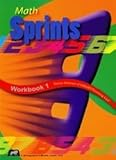#### Math Sprints Workbook 2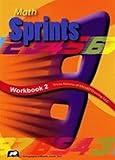#### Math Sprints Workbook 5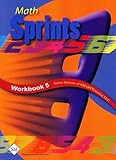#### Math Sprints Workbook 3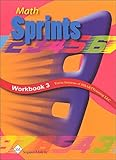#### Math Sprints Workbook 4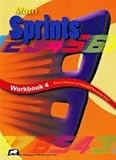Get a FREE subscription to Cathy's E-Newsletter

### My Lists

Save reviews to lists to guide curriculum choices. Register or log in to start your first list.

### Instant Key

• Need For Parent or Teacher Instruction: moderate
• Learning Environment: all situations